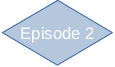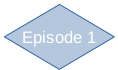Lesson 1a Algebra: Exploring area on a grid

# Lesson plan

Piagetian Level Learners Thinking and abstract of the activity
3 B
Mature
Formal
3A
Early
Formal
Expression in terms of generalised number '... so you can say that it's 2 x b x b + a x a''that one's a 2 by 3 paralellogram and a side-2 triangle so it's 12 plus 4'
2B*
Concrete
Generalisation
‘If it's 20 triangles
then you halve it to make 10. It could be base = 2 and side = 5.’Generalised summary without algebra ''side 2, area is 4; side 3, area is 9
2B
Mature
concrete
'if it's 1 row there are twice the number of triangles on the base''if the base is 1 the area is 2; if the base is 2 the area is 4' 'the 3 base has 6 triangles'
Middle
concrete
Episode 1
Focus on parallelogram

Episode 2
Focus on triangle

Episode 3
Focus on trapezium